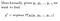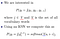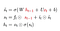# Encoder Decoder Models

This article covers the content discussed in the Encoder-Decoder Models module of the Deep Learning course offered on the website: https://padhai.onefourthlabs.in

Deep Learning is being used for a variety of tasks such as image classification, object detection, sequence learning problems, fraud detection, image captioning, natural language processing, speech recognition, summarization, and many other tasks. The key point is that in all of the tasks the model being used is some combination of the Fully Connected Neural Network(FCNN), Convolutional Neural Network(CNN), Recurrent Neural Network(RNN) and this combination of different networks is known as the Encoder-Decoder Model.

So, encoder just takes an input and it encodes the input and transmits it to the decoder. The decoder reads this encoded message and decodes the message from it. Typically, the encoder is a Neural Network and the decoder is also a Neural Network.

The problem of Language Modeling:

Given the ‘t — 1’ words, we try to predict the t’th word for example: let’s say we are given the input as ‘I am going to’ now based on this input the model might predict the next word as the ‘the’ and then the input would be ‘I am going to the’ and this time the model might suggest the word as ‘office’.Given the input y1, y2, ….., y(t-1); what is the likely word at the t’th time step, we compute the probability of all the possbile words and the one with the max probability value is returned

Given the input y1, y2, ….., y(t-1); what is the most likely word at the t’th output/time step, we compute the probability(using Softmax) of all the possible words and the one with the maximum value of probability is returned, argmax means return that word which has the maximum probability.

So, lets model this using RNN.

The intermediate state s1 and the final output y(which would be a probability distribution) would be computed as:

We already discussed in the article of RNNs about how we encode the input xo, as the input is going to be a sequence of words/characters, we encode the words/characters and then generate one hot vector all the words/characters in the input.

Let’s say the model gives the output as ‘I’ for the first input; now this word ‘I’ would act as the input at the 2nd time step and in a way the model’s output at any time step itself is serving as the input at the next time step so that’s the reason we use y1, y2, ……, y(t-1) in the equation of y*

Output at the first time step is ‘I’ and is denoted by y1, now the input at the next time step is also ‘I’ but this time we denote it is as x2 and the reason is that x2 represents the output y1 in one hot encoded form.

Now we compute the s2 using the same formula as:

We compute y2 as:

And this process continues like this

We pass on the output at the 2nd time step as the input at 3rd time step and the previous input(first two input) are captured in the hidden state s2(red box in the above image). And using this previous state as well as the current input, we compute the current state and from that, we compute the output. Hence, we can say that the output depends upon all the previous inputs(captured in the state vector) as well as the current input.

And this story continues all the way till the end

So, whenever it predicts <stop>(or <eos>), we know that now we don’t need to predict anything and give the entire sentence generated till now from y1 to yt(output at the final time step) as the final output.

Encoder Decoder ModelV(red underline) used in the first point refers to the set of all the vocabulary words and has nothing to do with the parameter V(blue underline) used in the second point

We want to compute the output as per the probability equation in the above image and as per that formula, we consider all the inputs from y1, y2, ….. all the way up to y(t-1) but on the right hand side of the same equation we are given this probability as dependent on st and some parameters. So, st has all the information of all the inputs till now i.e y1, y2, ……, y(t-1). So, the model has encoded all this information into the hidden state vector st. So, RNN acts as the encoder here, it gives us the blue vector st which is an encoding of all the inputs seen so far including the current input. And now once everything has been encoded into this blue vector st, we give it to the output layer which tries to decode the answer(next word in this case) from the input/encoded value and it does so mathematically using the probability distribution.

So, our RNN is the encoder and the simple feed-forward(we pass st to sigmoid after multiplying it with the parameters V and adding bias b to it) network is the decoder.

Since st has encoded all the input information y1, y2, ……., y(t-1), we can write

# Connecting Encoder-Decoder model to the Six Jars

Task: Sequence Prediction Task — Given the previous input y1, y2, y3, ……., y(t-1), our job is to predict yt. As the input is a sequence so the model that we will choose would most probably have RNN in it.

Data:

Example:

Let’s say we pass the first wordIndia’ to our model, the true distribution y1 would have all the mass on the word ‘officially’ and since this is the very first step so the parameters would not have the correct configuration so let’s assume that it gives us some distribution y1_hat.

Now our job is to define a loss function between y1 and y1_hat so that we can get a loss and the loss can be backpropagated and the parameters W, U, V get updated.

Now at the next time step, we feed in the input ‘officially’, the true distribution would have the entire probability mass (meaning value 1 for the true output and 0 for all other words in the distribution) on the ‘the’ word and from our model, again we get a predicted distribution from our model as y2_hat, we compute the loss and backpropagate through the model.

Model:

So, given some words we want to predict the next word so that’s the relation we are interested in, we want y as a function of x and instead of x which is from y1, y2, … to y(t-1), we encode all of that information in st; st in turn depends on xt(first equation in the above image) hence we can have st as the substitute for all the previous inputs. So, we have only these 2 equations in the model, at every time step we compute the hidden state representation and from that, we compute the probability distribution y and then we compute the loss and the loss would be the sum of the cross-entropy loss at every time step.

There would be say some ‘T’ time steps, at every time step we would have some loss value.

At the first time step, the model should predict India(first word in the training data taken in this case), so whatever is the loss at not predicting India, in a way we want to maximize the probability of the output as ‘India’(true class) given the <GO>(represents start of sequence) because y1, y2, ….., y(t-1) is just <GO> in this case, through loss function. At the next time step, we want to maximize the probability of y2 as the word ‘officially’ given the input as ‘India’ and we represent this input ‘India’ through encoded vector represented by the state. So, at every time step we have a classification problem and the loss function for a classification problem is the Cross-Entropy Loss(also called as Negative Log-Likelihood)(we take the log of the probability of the correct word at that time step as predicted by the model) and we sum this loss over all time steps and that becomes the overall loss function.

And once we have the Loss, we are going to use the Gradient Descent Algorithm with Backpropagation through time(as discussed in the case of RNN).

# A compact notation for RNNs, LSTMs, and GRUs

The main part in the RNNs is the state vector st and we compute state using the below equation:

Instead of writing st every time like this, we write it in a compact form as:

So, as per this compact form, st is computed from the RNN function which takes s(t-1) and xt as the input and the RNN function just maps to the original equation that we have, this is just the compact way of writing the same.

In the case of GRUs, we compute st as:

Here as per the second equation, st is compute from s(t-1) and st(~), however, st(~) in turns depends on s(t-1) and xt, so we can say that st depends on s(t-1) and xt and these acts as input to out function.

We write this in compact form as

And in LSTMs, we compute the state st, ht as:Red part denotes the input, so we 3 inputs and we produce output as st and ht.

The compact way of writing this is:

I have discussed the task of Image Captioning and Machine Translation using the Encoder Decoder Models in the following article:

https://medium.com/@prvnk10/encoder-decoder-model-for-image-captioning-e01c9392ea7f